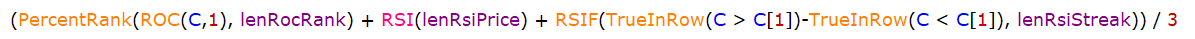Navigation: Realtest Script Language > Syntax Element Details > CRSI

Category

Indicator Functions

Description

Connors RSI Indicator

Syntax

CRSI(lenRocRank, lenRsiPrice, lenRsiStreak)

Parameters

lenRocRank - number of bars to use in the PercentRank(ROC(C,1), len) portion of the calculation

lenRsiPrice - length parameter for the price RSI portion of the calculation

lenRsiStreak - length parameter for the "streak" RSI portion of the calculation

Notes

This calculates the equivalent of the following formula:CRSI was added as a built-in function to improve calculation speed and enable "reverse CRSI" to be calculated by passing it to the TargetPrice function.